Prev: Directional Lights I Next: Dir. Light per Pixel

Time for the specular component of the OpenGL directional light. The lighting model used is the Blinn-Phong model, which is a simplification of the Phong model. We shall take a peek at the Phong model since it makes it easier to understand the Blinn-Phong model.

The Phong model says that the specular component is proportional to the cosine between the light reflection vector and the eye vector. The following image shows this graphically: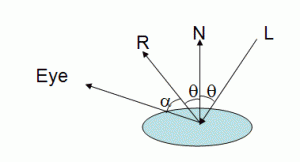L is the vector from the light to the vertex being shaded. N is the normal vector, and Eye is the vector from the vertex to the eye, or camera. R is the vector L mirror reflected on the surface. The specular component is proportional to the cosine of alpha.

If the eye vector coincides with the reflection vector then we get the maximum specular intensity. As the eye vector diverges from the reflection vector the specular intensity decays. The rate of decay is controlled by a shininess factor. The higher the shininess factor the faster the decay. This means that with a high shininess the bright spot caused by the specular component is smaller than with a low shininess value. Simply put, the shininess (a value between 0 and 128 in OpenGL) controls the size of the bright spot.

The formula for the reflection vector is as follows: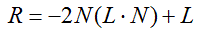And the specular component in OpenGL using the Phong model would be: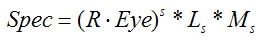Where the s exponent is the shininess value, Ls is the lights specular intensity, and Ms is the materials specular coefficient.

Blinn proposed a simpler and faster model, knows as the Blinn-Phong model that is based on the half-vector. The half-vector is a vector with a direction half-way between the eye vector and the light vector as shown in the following figure:The intensity of the specular component is now based on the cosine of the angle between the half vector and the normal.

The formula for the half-vector is much simpler than for the reflection vector: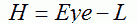Both vectors,Eye and L must be previously normalised, and H must also be normalized. The specular component in OpenGL using the Blinn-Phong model is: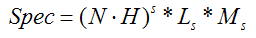This is the actual stuff as commonly used in the fixed pipeline of the graphics hardware. Since we want to emulate the OpenGL’s directional light, we’re going to use this last equation in our shader. There is a good news: OpenGL computes the half-vector for us! So the following snippet of code should do the trick:

```	/* compute the specular term if NdotL is  larger than zero */
if (NdotL > 0.0) {

// normalize the half-vector, and then compute the
// cosine (dot product) with the normal
NdotHV = max(dot(normal, gl_LightSource.halfVector.xyz),0.0);
specular = gl_FrontMaterial.specular * gl_LightSource.specular *
pow(NdotHV,gl_FrontMaterial.shininess);
}```

The full source of the shaders, in a Shader Designer project can be found in here.

 Prev: Directional Lights I Next: Dir. Light per Pixel

### 11 Responses to “Directional Lights II”

1.hi, i did the calculation for both specular formulas and i get 2 different results.
in fact, i noticed that the angle between R to EYE is twice the angle between N to H.
meaning that if N*H=cos(alpha) then: R*EYE = cos (2*alpha).
shouldn’t they be the same?
I’d be happy for clarification.

thanks
David

2.Is L normalize(-ftransform()) or -normalize(gl_ModelViewMatrix * gl_Vertex) , I just don’t know if I also have to consider the projection matrix.

•Hi Ramy,

L being the direction towards the light must be transformed to camera space (this is an option, we are transforming everything to camera space to perform the computations there). So, if the light is provided by the application in world space then the light must be transformed only by the View matrix. If the light is provided in local space then it must be transformed by the ViewModel matrix. If the light is already provided in camera space then there is no need to transform 🙂

The Projection matrix is not used in any of these scenarios.

Hope this helps.

3.Eye – L can sometimes be zero. Usually you want Spec = L_s * M_s in this case instead of the zero that results from Spec = (dot(N, H))^s L_s * M_s.

•Well, theoretically yes, Eye – L can be zero. But that implies that either the camera or the light are on the wrong side of the plane, and these situations are usually tested in the shaders before computing the specular component.

4.Eye and H should be normalized, right?

•Hi Blair,

Yes, thanks for spotting that omission. Fixed 🙂

5.code should look like
float tmp = pow(NdotHV,gl_FrontMaterial.shininess);
if ( NdotHV == gl_FrontMaterial.shininess && NdotHV == 0)
tmp = 1;

specular = (gl_FrontMaterial.specular* gl_LightSource.specular) * tmp;

6.If NdotHVis zero and gl_FrontMaterial.shininess is also zero, then pow function misbehaves and give unexpected result.

pow(NdotHV,gl_FrontMaterial.shininess)

7.The image after this sentence: “The half-vector is a vector with a direction half-way between the eye vector and the light vector as shown in the following figure” should probably be halfV.giv instead of eqhalfV.gif.

•Thanks. Bug fixed.

This site uses Akismet to reduce spam. Learn how your comment data is processed.